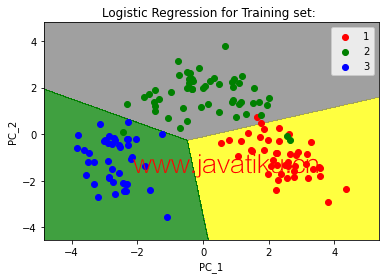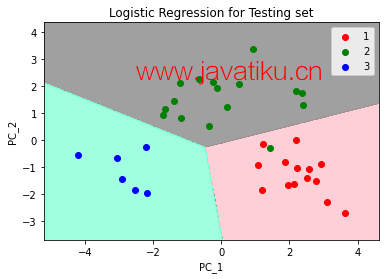# Python教程-用Python进行主成分分析（PCA）## 主成分分析的不同用途：

• PCA可用于查找数据中各个变量之间的相互关系。
• PCA可用于解释和可视化数据集。
• PCA还可用于可视化基因距离和不同群体之间的关系。
• PCA还通过减少变量的数量来简化分析。

## 主成分分析的目标是什么？

PCA的基本目标如下：

• PCA是一种无依赖方法，可用于从一组较多变量的属性空间减少到较少因子的数量。
• 它是一种降维技术，但不能保证降维后的因子是可解释的。
• 在PCA中，主要工作是从较多变量的集合中选择一组子集，该子集的原始变量与主要量的相关性最高。

## 使用Python进行PCA的步骤如下：

``````import numpy as nmp
import matplotlib.pyplot as mpltl
import pandas as pnd  ``````

``````DS = pnd.read_csv('Wine.csv')

# Now, we will distribute the dataset into two components "X" and "Y"

X = DS.iloc[: , 0:13].values
Y = DS.iloc[: , 13].values  ``````

``````from sklearn.model_selection import train_test_split as tts

X_train, X_test, Y_train, Y_test = tts(X, Y, test_size = 0.2, random_state = 0)  ``````

``````from sklearn.preprocessing import StandardScaler as SS
SC = SS()

X_train = SC.fit_transform(X_train)
X_test = SC.transform(X_test)  ``````

``````from sklearn.decomposition import PCA

PCa = PCA (n_components = 1)

X_train = PCa.fit_transform(X_train)
X_test = PCa.transform(X_test)

explained_variance = PCa.explained_variance_ratio_  ``````

``````from sklearn.linear_model import LogisticRegression as LR

classifier_1 = LR (random_state = 0)
classifier_1.fit(X_train, Y_train)  ``````

``LogisticRegression(random_state=0)``

``Y_pred = classifier_1.predict(X_test)  ``

``````from sklearn.metrics import confusion_matrix as CM

c_m = CM (Y_test, Y_pred)  ``````

``````from matplotlib.colors import ListedColormap as LCM

X_set, Y_set = X_train, Y_train
X_1, X_2 = nmp.meshgrid(nmp.arange(start = X_set[:, 0].min() - 1,
stop = X_set[: , 0].max() + 1, step = 0.01),
nmp.arange(start = X_set[: , 1].min() - 1,
stop = X_set[: , 1].max() + 1, step = 0.01))

mpltl.contourf(X_1, X_2, classifier_1.predict(nmp.array([X_1.ravel(),
X_2.ravel()]).T).reshape(X_1.shape), alpha = 0.75,
cmap = LCM (('yellow', 'grey', 'green')))

mpltl.xlim (X_1.min(), X_1.max())
mpltl.ylim (X_2.min(), X_2.max())

for s, t in enumerate(nmp.unique(Y_set)):
mpltl.scatter(X_set[Y_set == t, 0], X_set[Y_set == t, 1],
c = LCM (('red', 'green', 'blue'))(s), label = t)

mpltl.title('Logistic Regression for Training set: ')
mpltl.xlabel ('PC_1') # for X_label
mpltl.ylabel ('PC_2') # for Y_label
mpltl.legend() # for showing legend

# show scatter plot
mpltl.show()  ````````````from matplotlib.colors import ListedColormap as LCM

X_set, Y_set = X_test, Y_test

X_1, X_2 = nmp.meshgrid(nmp.arange(start = X_set[: , 0].min() - 1,
stop = X_set[: , 0].max() + 1, step = 0.01),
nmp.arange(start = X_set[: , 1].min() - 1,
stop = X_set[: , 1].max() + 1, step = 0.01))

mpltl.contourf(X_1, X_2, classifier_1.predict(nmp.array([X_1.ravel(),
X_2.ravel()]).T).reshape(X_1.shape), alpha = 0.75,
cmap = LCM(('pink', 'grey', 'aquamarine')))

mpltl.xlim(X_1.min(), X_1.max())
mpltl.ylim(X_2.min(), X_2.max())

for s, t in enumerate(nmp.unique(Y_set)):
mpltl.scatter(X_set[Y_set == t, 0], X_set[Y_set == t, 1],
c = LCM(('red', 'green', 'blue'))(s), label = t)

# title for scatter plot
mpltl.title('Logistic Regression for Testing set')
mpltl.xlabel ('PC_1') # for X_label
mpltl.ylabel ('PC_2') # for Y_label
mpltl.legend()

# show scatter plot
mpltl.show()  ``````